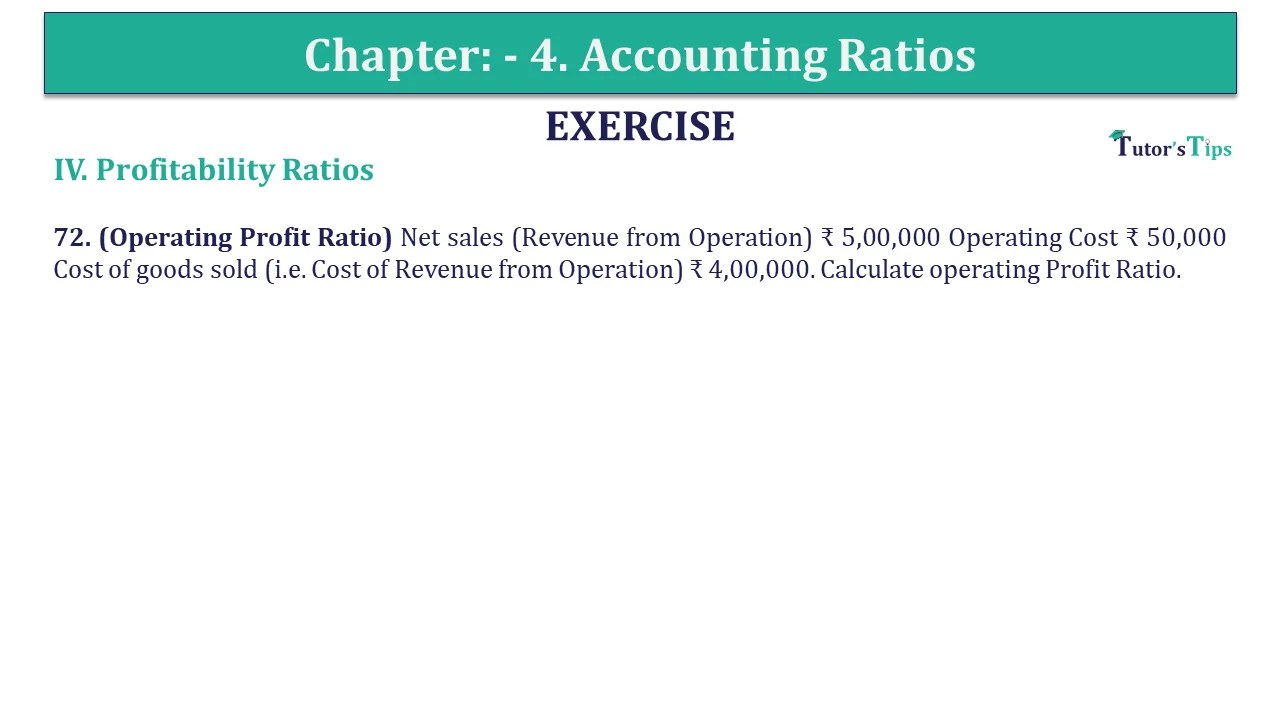# Question 72 Chapter 4 of +2-B – USHA Publication 12 ClassQ-72- CH-4 Book 2 - Usha Pub. +2 Book 2020 - Solution

Question 72 Chapter 4 of +2-B

IV. Profitability Ratios

72. (Operating Profit Ratio) Net sales (Revenue from Operation) ₹ 5,00,000 Operating Cost ₹ 50,000 Cost of goods sold (i.e. Cost of Revenue from Operation) ₹ 4,00,000. Calculate operating Profit Ratio.

## The solution of Question 72 Chapter 4 of +2-B: –

 (a) Operating Profit Ratio = ₹ 50,000 X ₹ 2,00,000 ₹ 5,00,000 = 10%
 Operating Profit = Net Sales – Cost of goods sold – Operating Cost = ₹ 5,00,000 – ₹ 4,00,000 – ₹ 50,000 = ₹ 50,000

Also, Check out the solved question of previous Chapters: –